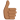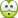# Special Relativity Exercise (A.P. French)

• alejandromeira
In summary, the correct answer for question c) is that the relative speed between the two reference systems is 0.9155c. However, this is not the only possible answer as it depends on the direction of motion and the relative orientation of the two reference systems. This is known as the indeterminacy of simultaneity in special relativity.

#### alejandromeira

Thread moved from the technical forums, so no Homework Template is shown
I just solved an exercise in the special relativity book of A.P. French. I would like you to tell me if the answer is correct. Yes I suposse is a very easy exersice.
I hope you can help me, because I really like learn relativity and cosmology, but I have not had teachers of this, and I am completely self-taught in the subject.

Exercise.

In a certain reference system it is observed that a particle has a total energy of 5GeV, and an P of 3GeV/c, (that is to say cP, which has units of energy, is 3GeV).

a) What is the energy of this particle in a system where P is 4GeV/c ?
b) What will be your mass at rest in uma?
c) What is the relative speed of the two reference systems?

My atempt of solution.
Question a)
Ok, there are two systems of reference
System 1: E_1, P_1
System 2: E_2 (unknow), P_2
I apply the relativistic invariant for the four moment energy ; ##E_0^2=E^2-c^2p^2##, then: $$E_1^2-c^2p_1^2=E_2^2-c^2p_2^2$$
I make sustitution of terms: E_1=5GeV; P_1=3GeV/c; E_2=?; P_2=4GeV/c$$5^2-c^2(\frac 3 c)^2=E_2^2-c^2(\frac 4 c)^2$$ I solve and the result is: ##E_2=4\sqrt2 \text { GeV}##

Question b)
I use the reference system 2 for compute te invariant energy-momentum $$E_0^2=E_2^2 - c^2p^2$$ I put the values into the above equation: $$E_0^2=(4\sqrt 2)^2-c^2(\frac 4 c)^2$$ Solution is ##E_0=4\text{GeV}##
We can use the conversión factors and achieve a rest mass ##m_0=4.28\text{u}##, (atomic units of mass)

Question c)
For easyest calculations I use masses in GeV in this question.
In the system 2 particle have a rest mass m_0 of 4GeV, but
In ths system 1 particle have a mass of 5GeV. Then $$\frac{ m_1} {m_0}=\gamma$$$$\frac{ 5} {4}=\gamma=1.25$$
Then ##\gamma=\frac{1}{\sqrt{1-\beta^2}}##.
##\beta=0.6##, and then ##v=0.6c##
******************************************************************************************************************
Ok indications are welcome, thanks a lot.

(a) and (b) are correct. In (c) you are not asked for the velocity relative to the rest frame of the particle, you are asked for the relative velocity between the different inertial frames. This cannot be answered without additional assumptions (such as the particle moving in the x-direction in both frames).

Also
alejandromeira said:
In the system 2 particle have a rest mass m_0 of 4GeV
the rest mass (or just mass, we really do not talk about "relativistic mass" anymore) is invariant, i.e., it is the same in all frames.

Ok, then how can I solve question c)
I'm a teacher in a High School. And I work in relativity only because I like to learn about this subject. Then you can say me the answer without problem, I'm not doing my homework for tomorrow!(the post was moved here)

alejandromeira said:
Then you can say me the answer without problem, I'm not doing my homework for tomorrow!
We treat all schoolwork questions the same here at the PF. Whether it is for self study or for an assignment for class. We require that you show your work and try to figure out the problems -- we are not allowed to give answers to schoolwork-type questions here.alejandromeira said:
Ok, then how can I solve question c)

Have you heard of the "energy-momentum transformation"?

•alejandromeira
First of all, I'm sorry if I did not follow any rules. Rules are for all people, of course! You can be sure that I have my honesty : -)

PeroK said:
Have you heard of the "energy-momentum transformation"?

I suposse transform with a Lorentz transformation, like time- space. Is it?

•berkeman
alejandromeira said:
First of all, I'm sorry if I did not follow any rules. Rules are for all people, of course! You can be sure that I have my honesty : -)

I suposse transform with a Lorentz transformation, like time- space. Is it?

Yes, it should be in AP French's book somewhere.

Ok, I need to make Tle lorentz transformation between E and E'! How I did not realize that!
Thank you very much.A (much) more convenient way (if you are familiar with it) than applying the Lorentz transformation is to use the Minkowski inner product between the 4-momenta.

•alejandromeira
Orodruin said:
A (much) more convenient way (if you are familiar with it) than applying the Lorentz transformation is to use the Minkowski inner product between the 4-momenta.
Ok, thanks, this for tomorrow, for today is adequate for me.I have made this transformation: $$E'=\frac{E-p_x·v}{\sqrt {1-\beta^2}}$$E=5 GeV; Px=3/c GeV; E'=4·sqr(2) GeV; $$4\sqrt {2}=\frac{5-\frac 3 c·v}{\sqrt {1-\beta^2}}$$$$4\sqrt {2}=\frac{5-3\beta}{\sqrt {1-\beta^2}}$$
Solving: $$41\beta^2-30\beta-7=0$$$$\beta^2-0.731\beta-0.17=0$$
$$\beta=\frac {0.731\pm\sqrt{0.731^2+4·0.17}}{2}=\frac{0.731\pm1.10}{2}=0.9155$$and ##v=0.9155c##

It's ok?

No. You cannot just throw away one of the roots. (This is part of the indeterminacy I already mentioned.)

Ok Orodruin, I will think in these questios tomorow in the afternoon, today is too later.
I also think in calculate whit the rest mass the two velocities of the two reference frames respect to the rest frame, and then whit the formula or relatives velocities calculate it betwen the two, but I don't now if this procedure are ok.
Ok is too late I go to sleep... zzzzzz

Orodruin said:
A (much) more convenient way (if you are familiar with it) than applying the Lorentz transformation is to use the Minkowski inner product between the 4-momenta.
Ok, I do not know very well what you mean, because my knowledge is very limited, but I have tried this, looking for the angle between the 4-momenta, in the hope that this angle are related with beta. Units are in GeV.
## \vec {p_1}=\begin{pmatrix}E_1\\p_1·c\end{pmatrix}=\begin{pmatrix}5\\3\end{pmatrix};\vec{p_2}=\begin{pmatrix}E_2\\p_2·c\end{pmatrix}=\begin{pmatrix}4·\sqrt2\\4\end{pmatrix}##
Minkosky metric and inner product: $$\eta_{ab}=\begin{pmatrix}-1&0\\0&1\end{pmatrix}; \vec X·\vec Y=\eta_{ab}·X^a·Y^b$$
We calculate, only terms in that a=b do not vanishing, then is not too hard:$$\vec {p_1}·\vec {p_1}=p_1^2=-1·5^2+1·3^2; p_1=\sqrt{-16}$$$$\vec {p_2}·\vec {p_2}=p_2^2=-1·(4·\sqrt2)^2+1·4^2; p_2=\sqrt{-16}$$
Ok this seems to be invariant. Now we calculate P_1·P_2.$$\vec {p_1}·\vec {p_2}=-1·5·4\sqrt2+1·3·4=-16,28$$
But now I have a problem, because if I try to calculate the angle:$$cos(\theta)=\frac{\vec p_1·\vec p_2}{p_1·p_2}=\frac{-16,28}{-16}>1$$
Then a this point I don't understand anything. Then I need your help.
And Orodruin do you refers to this calculations when you says the QUOTE above?

"Angles" work differently in Minkowski space. What you are looking for is the rapidity between the frames. Due to the hyperbolic nature, the rapidity between two 4-vectors ##P_1## and ##P_2## is given by
$$\cosh(\theta) = \pm \frac{P_1 \cdot P_2}{\sqrt{P_1^2 P_2^2}}$$
rather than ##\cos(\theta)##. The ##\pm## depends on the sign convention you are using for the inner product, most particle physicists would use ##+## in the time component.

Edit: Also, the relation between the rapidity and the relative speed is
$$\beta = \tanh(\theta).$$

•alejandromeira
Wow!Ok. Today I have an institutional act, and I will not be able to continue this work until tomorrow.

I've used -1 for temporal component, but from now one I will use the +1 sign.Ok, another question, if I use the sign +1 in the minkowsky metric then also is + in the above equation?
I'm not to much accustomed to the hyperbolics trigonometrics functions, and will need to buy a handle calculator with them. Those expressions of exponentials and logarithms are tedious for handle.

Thanks a lot! I will post the answer tomorrow, when I have finished the calculations.

alejandromeira said:
I'm not to much accustomed to the hyperbolics trigonometrics functions, and will need to buy a handle calculator with them. Those expressions of exponentials and logarithms are tedious for handle.
They do not differ that much from the usual trigonometric counterparts apart from the appearance of some relative minus signs. In particular, a minus sign in the hyperbolic one as compared to the trigonometric one:
$$\cosh^2(\theta) - \sinh^2(\theta) = 1.$$
In particular, this means that
$$\tanh(\theta) = \frac{\sinh(\theta)}{\cosh(\theta)} = \frac{\sqrt{\cosh^2(\theta) - 1}}{\cosh(\theta)},$$
which is all you need here.

Ok, then for calculate betha, with the above results, we do: $$cosh(\theta)=\frac{\vec p_1·\vec p_2}{p_1·p_2}=\frac {-16.28}{ -16}=1.0175$$ and:
$$\beta=tanh(\theta)=\frac{\sqrt{[cosh(\theta)]^2-1}}{cosh(\theta)}=\frac{0.1879}{1.0175}=0,1847$$$$v=0.1847·c$$
I suposse I'ts ok : -)

So this reproduces the (absolute value of the) result that you got from using the ##-## root of your previous second order equation. How would you reproduce the ##+## root?

Ok, the bull is not dead yet...Well, since you found two solutions before, you should ask yourself why you found two solutions.

$$sinh(\theta)=\pm\sqrt{[cosh(\theta)]^2-1}$$
is a square equation, then there are two solutions. Is it?

No, that is not it. That is just a redefinition of the positive direction of ##\theta##. The difference comes (much) earlier and has to do with your assumptions when you derived your original second order equation in relation to your assumptions when you wrote down the 4-momenta of the particles.

Ok, I've been thinking about that question for an hour, but I'm sorry, I think my current knowledge is not enough to answer it

alejandromeira said:
Ok, I've been thinking about that question for an hour, but I'm sorry, I think my current knowledge is not enough to answer it
I believe they should be, so go back to look at your assumptions.

Ok, I'm going to check everything, from the beginning.
In the Lorentz transforms, I understand, E, E ', + Px · V, -Px · v, and the labels in the vectors ... All that can lead to different results.

All this will take me time, maybe one day. Be patient, when I have a result I will post it.
And lots of thanks for your help!

Well, I just took a walk to clear my head, and when I got back it was much better.

I think the problem is ok. Also, this question only can be solved if the two systems moving along X-axis.
First of all, when I wrote the lorentz equations there was an error in a sign. This changes the direction of the spatial moment.
The spatial moments are, 3/c and 4/c but these can go:
A) (rigth and right) or
B) (rigth and left)

TYPE A (same direction).
Then the energy momentum vectors are: $$p_1=\begin{pmatrix}5\\3\end{pmatrix};p_2=\begin{pmatrix}4·\sqrt(2)\\4\end{pmatrix}$$ and it is the above discussion$$\beta=0.1847$$

TYPE B (oposite direction)
In this option energy momentum vectors are:$$p_1=\begin{pmatrix}5\\-3\end{pmatrix};p_2=\begin{pmatrix}4·\sqrt(2)\\4\end{pmatrix}$$
And then I made the calculations and give me:$$\beta=0.9175$$
Thats is the same result than in the wrong sign of the lorentzian method.
#######################################################################

Now I'm tired, but tomorrow I'll do a check. As I have the mass at rest, I will calculate the speeds with respect to the reference system at rest, and then I will use the law of adding speeds, in both cases (+, +) and (-, +), to see if this way also works.

Ok, I don't know if there are some error in the calculation, I think all i'ts ok.

alejandromeira said:
Also, this question only can be solved if the two systems moving along X-axis.
Well, as you have discovered, you have an ambiguity even then. In general, the ambiguity in direction will result in any relative speed between the values you got (note speed, not velocity).

Ok, l'm happy, tomorrow l will go to make the adittion of velocities for get moore practice in this problems. ThanksWell, I've made the addittion of velocities, and all calculations are ok. I use te formula: $$U_1=\frac{V_2-V_1}{1-V_1·V_2/c^2}$$ were:
U1= measured velocity of particle 2 in the 1 particle. (relative velocity of 2 measured in 1)
V1; V2= Velocity of particles 1 and 2 respect to the rest frame.
For that this formula works well one need to put the components of the velocity V1,V2, with theirs signs respec to te X axis.
(Is the formula for adition of velocities that I better handle)

Ok problem solved! Thanks for your patience and encouragement.